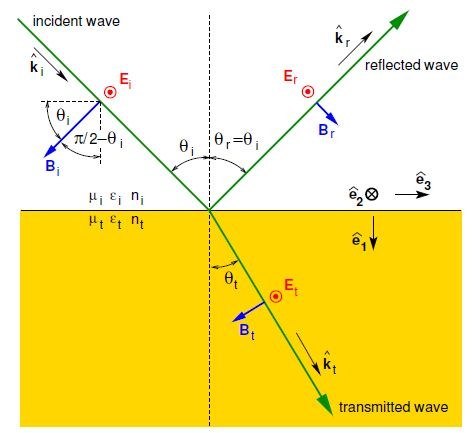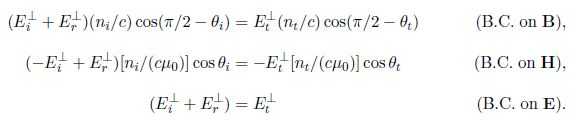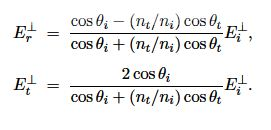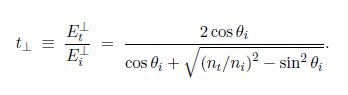# Amplitude Reflection and Transmission Coefficients for Perpendicular Polari-sation

Amplitude reflection and transmission coefficients for perpendicular polari-sationFigure: EM field geometry for perpendicular (σ, s) polarisation. The electric field is shown pointing out of the page.

The EM field geometry for perpendicular (σ, s) polarisation is shown in Figure. In this case, E • e1 = 0, E • e3 = 0 and B • e2 = 0, and so the three simultaneous equations remaining areSolving simultaneous Equations we obtain;Using Snell’s law nt sin θt = ni sin θi from which;

cos θt = √ (1 – ni2 / nt2 sin2 θi)

we arrive at the amplitude reflection coefficient for perpendicular polarisation,and the amplitude transmission coefficient for perpendicular polarisation,